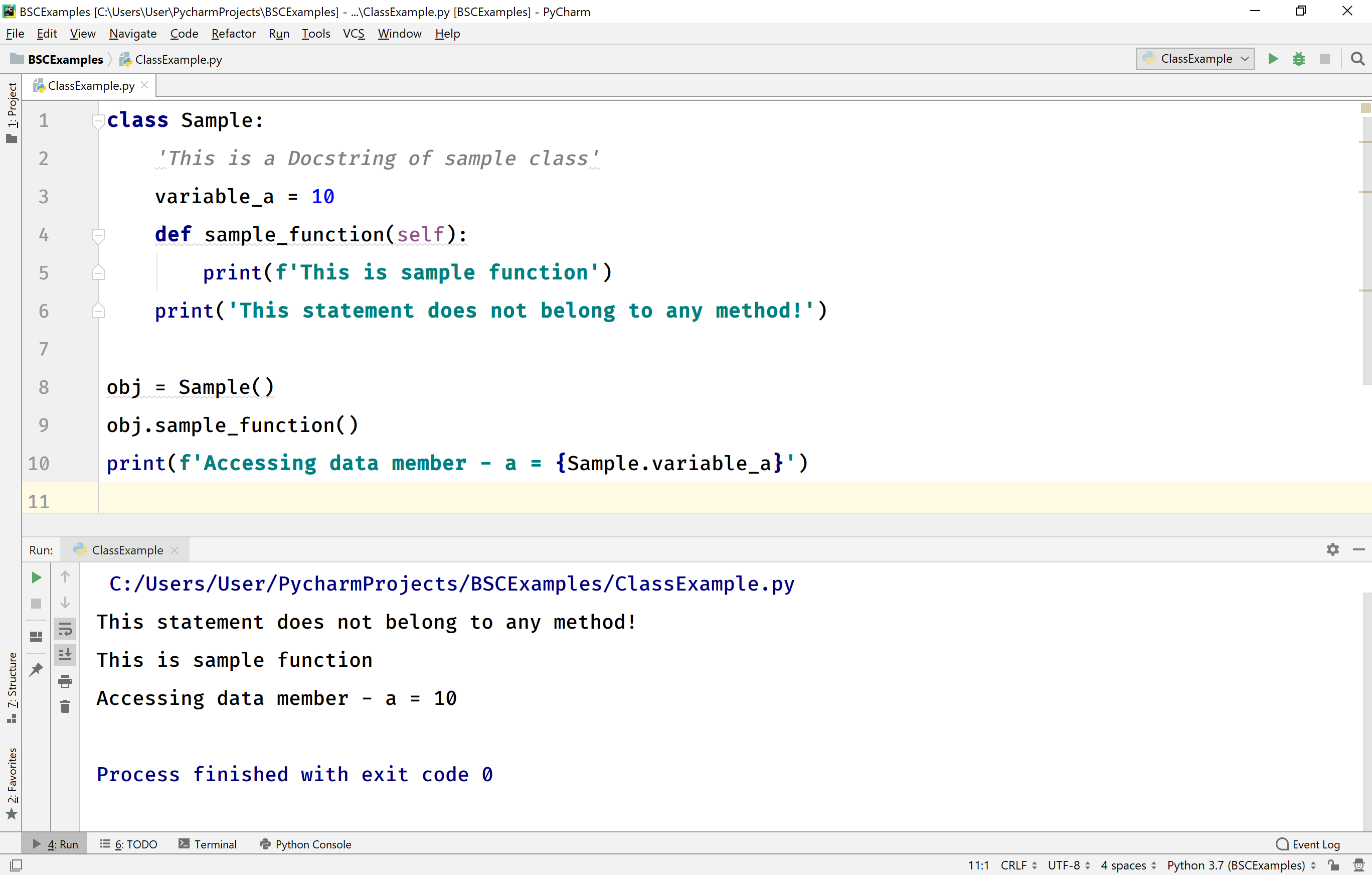The perfect place for easy learning...

×

# Python Class and Object

The Python is an object-oriented programming language from its beginning. Almost everything in Python implemented using object concept. The Python support all the features of OOP.

A class is a "blueprint" or "prototype" to define an object. Every object has its properties and methods. That means a class contains some properties and methods.

# Creating Class

In Python, we use the keyword class to create a class. The general class definition looks like the following.

Syntax
``````class ClassName:
'Docstring - An optional documentation string'
statement_1
statement_2
...
statement_n``````

In the above syntax, the class is the keyword used to define a class. ClassName can be any user-defined name but we must obey the naming rules. The Docstring is an optional documentation string used to describe the class. And the statement can be any statement.

The docstring of a class is accessed using ClassName.__doc__.

The class may contain attributes like data members (variables), code members (methods), and also any Python statements. When a class contains Python statements directly (means they do not belong to any method), they will be executed normally in the class.

# Creating Object

An object is a variable of class type. An object of a class is also known as an instance of a class. All the members of a class are accessed using an object of that class. We use the following syntax to create an object.

Syntax
``object_name = ClassName(arguments)``

Consider the following example.

Example
``````class Sample:
'This is a Docstring of sample class'
variable_a = 10
def sample_function(self):
print(f'This is sample function')
print('This statement does not belong to any method!')

obj = Sample()
obj.sample_function()
print(f'Accessing data member - a = {Sample.variable_a}')
``````

When we run the above example code, it produces the following output.In the above example, we have created a class called Sample with a data member (a), a member function (sample_function()), and a Python statement. We also created an object (obj) of the Sample class.

# Accessing Class Members

In Python, we use a dot (.) operator to access the members of a class. In the above example, we have used the following statements to access the sample_function() member function and a data member of Sample class.

Example
``````obj.sample_function()
print(f'Accessing data member - a = {Sample.variable_a}')
``````

In Python, the data members of a class need not be declared like local variables. We can add, modify, and delete data members at any time.

In the above example, there is only one data member (variable_a) but we can add new data members to the class at any time. A new data member is added to the class when it is assigned with a value. Let's add a new data member called variable_b. The following code adds variable_b to the class Sample.

Example
``````obj.variable_b = 100
print(f'Newly added data member variable_b = {obj.variable_b}')
``````

In Python, the member functions must define in the class. We can't add member functions later.

# Members Objects

In Python, we can create an object of a member function. The same object is used to call the member function. The following code creates an object method_obj for the member function sample_function(), and it is called used the same object.

Example
``````method_obj = obj.sample_function()
method_obj()
``````

# self Parameter

In Python, every method of a class must have the first parameter as self. Here, the self parameter refers to the current object being used to call that method.

However, it does not have to be named self, we can call it whatever we like, but it has to be the first parameter of any function in the class.

Consider the following example code.

Example
``````class Sample:
i = 10
def __init__(self, x):
self.x = x

def myFun_1(self):
print(f'This if myFun_1 and self.x = {self.x}')

def myFun_2(xyz): # Here xyz is used instead of self
print(f'This if myFun_2 and xyz.x = {xyz.x}')

obj = Sample(10)
obj.myFun_1()
obj.myFun_2()
``````

In the above example, the method myFun_1() has defined with self parameter and the method myFun_2() has defined with xyz. Here both the parameters refer to the current object being used to call the corresponding method.

When we run the above example code, it produces the following output.